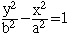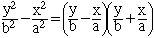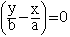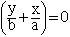Name: Carla Level of Question: Secondary (12) Asking question: Student Find the equation of the hyperbola centered at (0,0) with vertices at (0,3) and (0,-3) that is asymptotic to the lines y=+-5x. Find the equation of a hyperbola in standard position foci (1,5) and (1,7) and eccentricity 2. Thank you for your help and effort. Have a nice holiday and New Year!!! Thanks again!!! Hi Carla, For problem 1 the center being (0,0) and the vertices on the y-axis means that the hyperbola has the formIn this equation when x = 0, y2 = b2, that is y = +-b. But you know that (0,3) and (0,-3) are on the graph and hence b = 3. I remember that to find the asymptotes of the hyperbola in standard form you factor the left side as a difference of squares.The two asymptotes are thenandThis should allow you to find a. Cheers Penny Go to Math Central